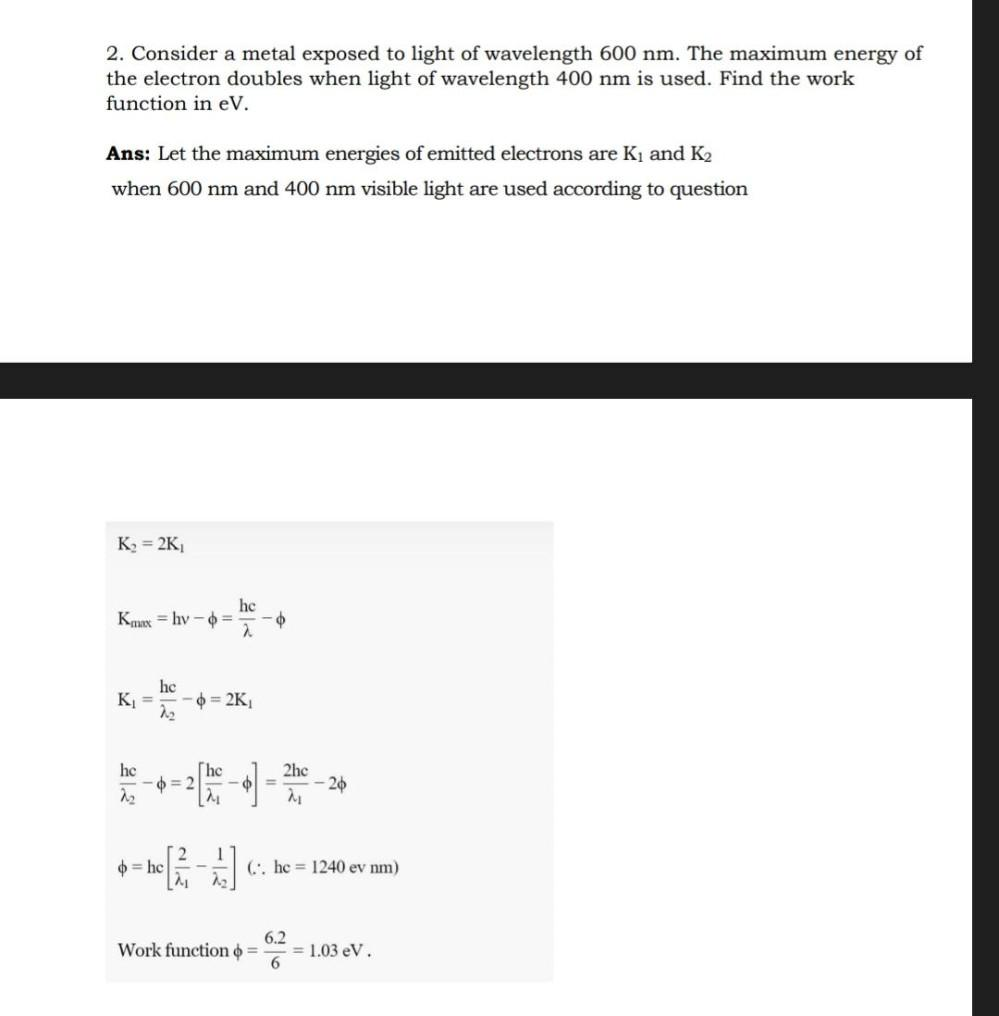Question:

# 2. Consider a metal exposed to light of wavelength 600 nm. The maximum energy of the electron doubles when light of wavelength 42. Consider a metal exposed to light of wavelength 600 nm. The maximum energy of the electron doubles when light of wavelength 400 nm is used. Find the work function in ev. Ans: Let the maximum energies of emitted electrons are Ky and K2 when 600 nm and 400 nm visible light are used according to question K2 = 2K hc Kmax = hy - 0 = 2 hc K= 22 = 2K K. - her - - - 2 - 4) - Film - 2 4-[3 ] hc 22 o = hc - 21 2hc - 21 2 1 0 = hc (::. hc = 1240 ev nm) 22 6.2 Work function o = 6 = 1.03 eV.